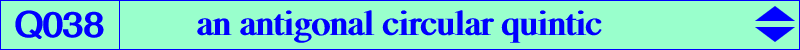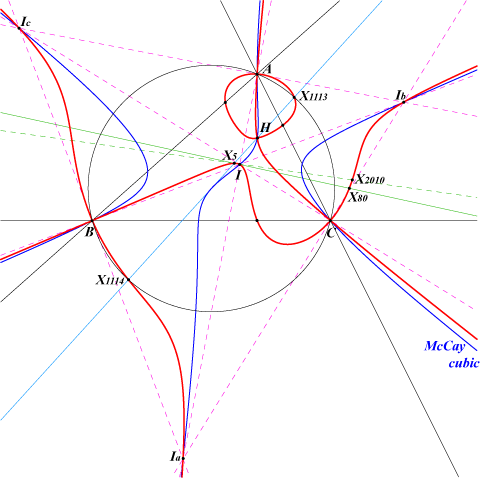too complicated to be written here. Click on the link to download a text file.X(1), X(4), X(5), X(80), X(1113), X(1114), X(1263), X(2009), X(2010) Ia, Ib, Ic : excenters Ha, Hb, Hc : feet of altitudes points at infinity of the McCay cubic extraversions of X(80) X5-OAP points, see also Table 53.Q038 is the isogonal transform of Q023. It is an antigonal circular quintic with three real asymptotes parallel to those of the McCay cubic. It has four double points namely A, B, C (with perpendicular nodal tangents) and H (with nodal tangents parallel to the asymptotes of the Jerabek hyperbola). The tangents at Ha, Hb, Hc concur at X(5), the nine-point center. K026 is the locus of points whose polar conic in Q038 is a rectangular hyperbola. In other words, the cubic is the Laplacian of the quintic.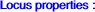Q038 is the locus of point Q such that : Q, its antigonal and X(5) are collinear (see generalization 2 below). there is a (non-isogonal) circum-strophoid with node Q passing through H. For example, if Q = H, the strophoid is K025, the Ehrmann strophoid. See CL038. there is a nodal circum-stelloid with node Q and asymptotes parallel to those of the McCay cubic. See K028 (Q = X4), K054 (Q = X5) for example. See also McCay Stelloids. Q and its isogonal conjugates with respect to the cevian and pedal triangles of Q are collinear. ABC and QaQbQc are perspective where Qa, Qb, Qc are the reflections of H about the lines AQ, BQ, CQ respectively (together with the line at infinity). Compare with Q023, locus property 3. ***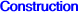A variable line (L) passing through O is the isogonal transform of a rectangular circum-hyperbola (H). The conjugated diameter of (L) in (H) meets (H) at two points on the quintic Q038. Note that this conjugated diameter is actually the line passing through X(5) and the center of (H), this latter point being the midpoint of H and the isogonal conjugate of the infinite point of (L), a point on (H) and on the circumcircle. In other words, for P on the nine point circle, the line X(5)P meets the rectangular circum-hyperbola passing through the reflection of H in P at two points on Q038. ***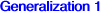Let P be a finite fixed point. A variable line (L) passing through P is the isogonal transform of a circum-conic (C) passing through the isogonal conjugate P* of P. The conjugated diameter of (L) in (C) meets (C) at two points on a circum-quintic denoted by Q(P). Q(P) contains P*, the in/excenters, the vertices of the cevian triangle of P*. The tangents at these three latter points concur for P on the Stammler hyperbola which is the polar conic of O in the McCay cubic. A, B, C and P* are four nodes on the curve. The nodal tangents at P* are perpendicular when P lies on the McCay cubic K003. Q(P) meets the line at infinity at 5 points : three are the infinite points of pK(K, P) – the isogonal pivotal cubic with pivot P – and the remaining two points are the infinite points of the circum-conic with center the complement of P*. Q(P) meets the circumcircle (O) at A, B, C (counting for six) and four other points lying on the rectangular hyperbola (Ho) passing through O, P*, the intersection of the Euler line and the trilinear polar of the X(110)–isoconjugate of P and whose asymptotic directions are those of the isogonal transform of the line OP. When P = O, (Ho) decomposes into the Euler line and the line at infinity since Q(O) is the only quintic passing through the circular points at infinity. (Ho) is a diagonal conic for Q = G. It is the union of two perpendicular lines for Q on K028, the third Musselman cubic. ***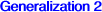Let P be a fixed point. The locus of M such that P, M and the antigonal of M are collinear is a circular circum-quintic Q2(P) passing through H, P, the antigonal P' of P, the vertices Ha, Hb, Hc of the orthic triangle. A, B, C, H are nodes on Q2(P). Further properties : When P = H, Q2(P) splits into the circumcircle and the altitudes of ABC. When P lies on the line at infinity, Q2(P) is bicircular. If P lies on the nine point circle, Q2(P) splits into the rectangular circum-hyperbola through P and the circular cubic pK(H x gaP, H) where gaP is the isogonal conjugate of the anticomplement aP of P. This point gaP is the isopivot and lies at infinity. The singular focus is the antipode of P on the nine point circle. These cubics are actually the isogonal circular pKs of the orthic triangle. More details in CL019. In all other cases, Q2(P) meets the line at infinity at the same points as the cubic pK(X6, aP) where aP is the anticomplement of P, Q2(P) meets the circumcircle again at two points on the line HP. The nodal tangents at H are perpendicular if and only if P lies on the Euler line and, in this case, these are parallel to the asymptotes of the Jerabek hyperbola. The nodal tangents at A, B, C are perpendicular if and only if P = X(5), giving the cubic Q038. The tangents at Ha, Hb, Hc pass through P.